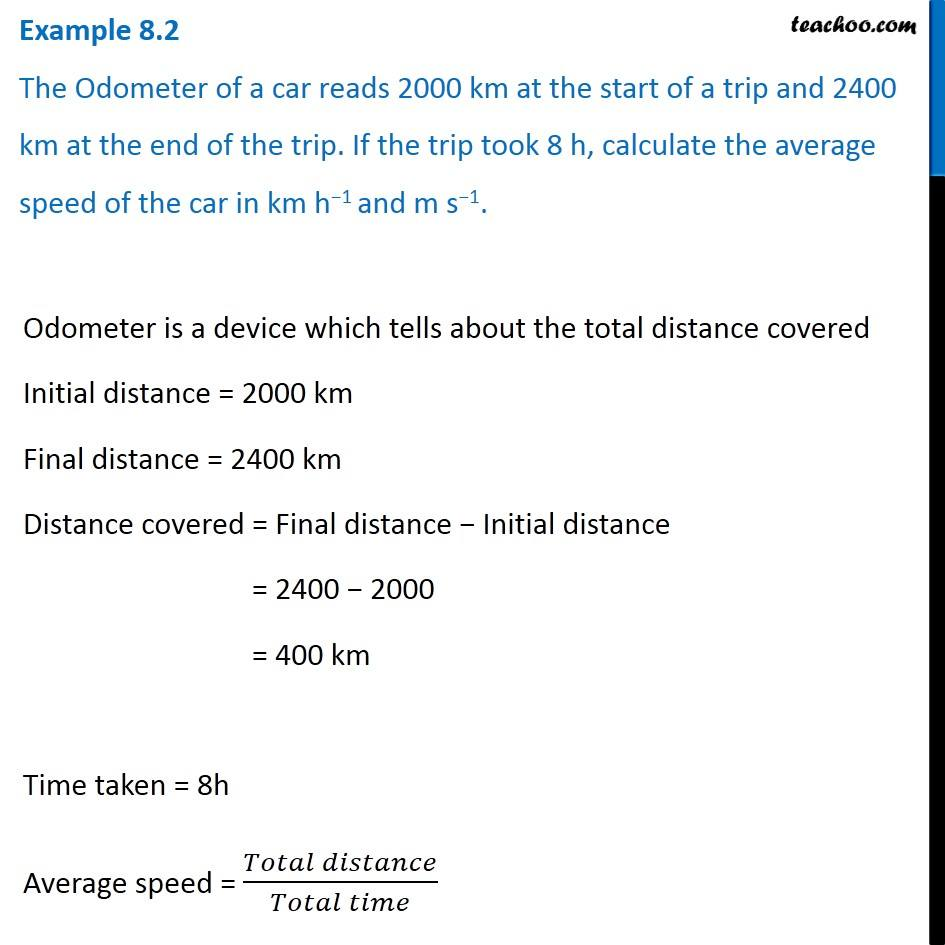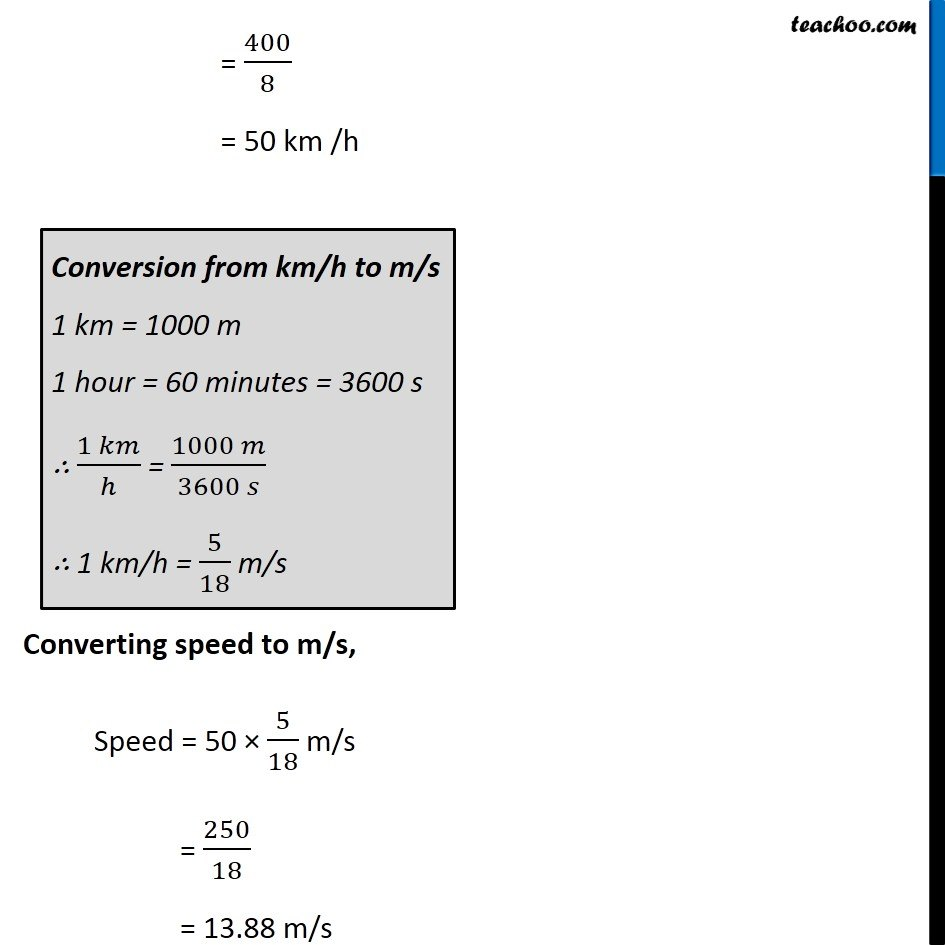Examples from NCERT Book

Class 9
Chapter 8 Class 9 - MotionLearn in your speed, with individual attention - Teachoo Maths 1-on-1 Class

### Transcript

Example 8.2 The Odometer of a car reads 2000 km at the start of a trip and 2400 km at the end of the trip. If the trip took 8 h, calculate the average speed of the car in km h−1 and m s−1. Odometer is a device which tells about the total distance covered Initial distance = 2000 km Final distance = 2400 km Distance covered = Final distance − Initial distance = 2400 − 2000 = 400 km Time taken = 8h Average speed = (𝑇𝑜𝑡𝑎𝑙 𝑑𝑖𝑠𝑡𝑎𝑛𝑐𝑒)/(𝑇𝑜𝑡𝑎𝑙 𝑡𝑖𝑚𝑒) = 400/8 = 50 km /h Conversion from km/h to m/s 1 km = 1000 m 1 hour = 60 minutes = 3600 s ∴ (1 𝑘𝑚)/ℎ = (1000 𝑚)/(3600 𝑠) ∴ 1 km/h = 5/18 m/s Converting speed to m/s, Speed = 50 × 5/18 m/s = 250/18 = 13.88 m/s